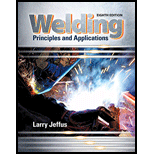Chapter 13, Problem 10R### Welding: Principles and Applicatio...

8th Edition
Larry Jeffus
ISBN: 9781305494695

#### Solutions

Chapter
Section### Welding: Principles and Applicatio...

8th Edition
Larry Jeffus
ISBN: 9781305494695
Textbook Problem
22 views

# Referring to Table 13-1, answer the following a. What would the range of the feed speed be for an amperage of 150 at 25 volts for an EM70-T 0.035-in. (09-mm) electrode? b. What would the approximate amperage be for an E70T-5 0045-in. (IQ-mm) electrode if it is being fed at a rate of 200 in. per minute (508 cm per minute)?

To determine

(a)

The range of feed speed.

Explanation

Given:

Amperage = 150

Voltage = 25volts

Now,

With the reference to the table 13.1 textbook,

To determine

(b)

The range of feed speed.

### Still sussing out bartleby?

Check out a sample textbook solution.

See a sample solution

#### The Solution to Your Study Problems

Bartleby provides explanations to thousands of textbook problems written by our experts, many with advanced degrees!

Get Started

#### Find more solutions based on key concepts